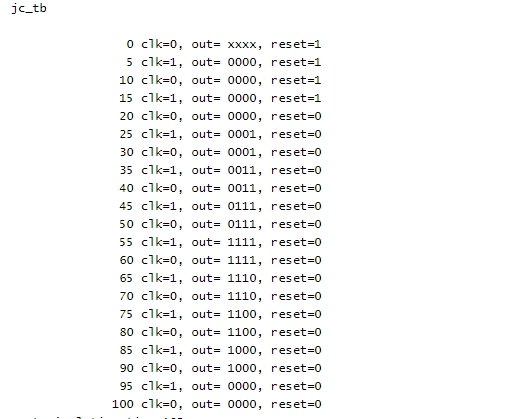# Johnson Counter Verilog Code | Verilog Code of Johnson Counter

#### Verilog Implementation of Johnson Counter

In this post we are going to share the Verilog code of Johnson counter. As we you know, Johnson counter is a counter that counts 2N states if the number of bits is N.  Here we are implementing it in HDL such as Verilog. The Verilog implementation of Johnson Counter is given below.

##### Johnson Counter Verilog Code
```///////Verilog Code Johnson COunter //////

module johnson_counter( out,reset,clk);
input clk,reset;
output [3:0] out;

reg [3:0] q;

always @(posedge clk)
begin

if(reset)
q=4'd0;
else
begin
q<=q;
q<=q;
q<=q;
q<=(~q);
end
end

assign out=q;
endmodule

//////End////```

##### The Testbench is given below.
```///////Test Bench //////

module jc_tb;
reg clk,reset;
wire [3:0] out;

johnson_counter dut (.out(out), .reset(reset), .clk(clk));

always
#5 clk =~clk;

initial begin
reset=1'b1; clk=1'b0;
#20 reset= 1'b0;
end

initial
begin
\$monitor( \$time, " clk=%b, out= %b, reset=%b", clk,out,reset);
#105  \$stop;
end

endmodule

/////End///////////```

### The simulation result of johnson counter verilog code is given below.View all posts by admin →